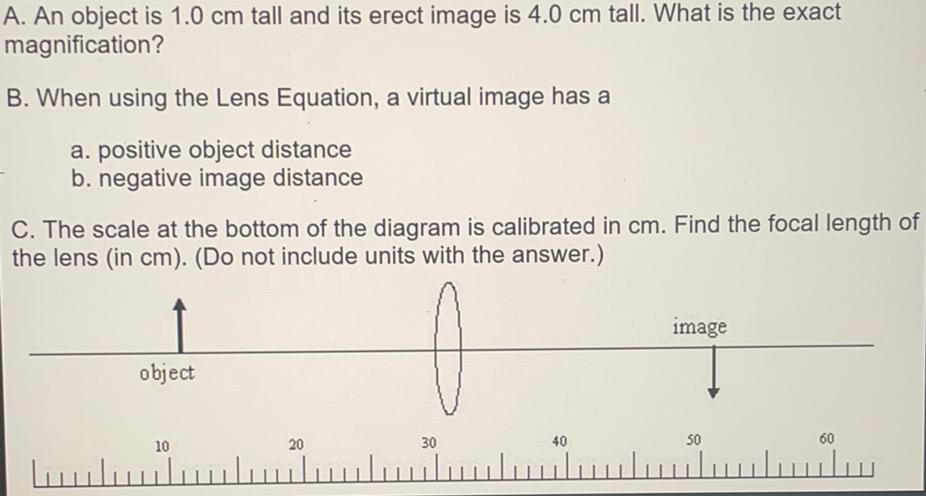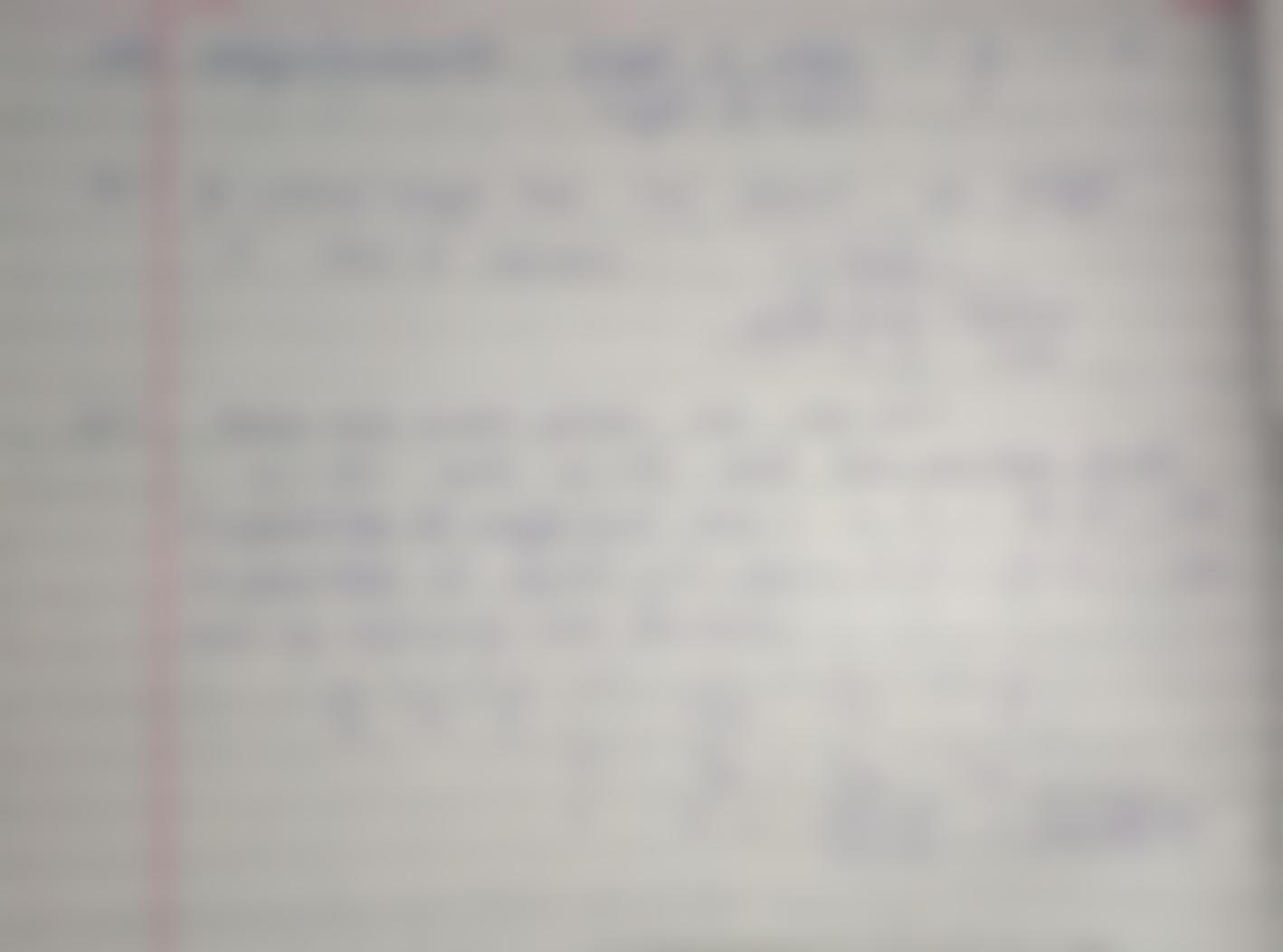Question:

# A. An object is 1.0 cm tall and its erect image is 4.0 cm tall. What is the exact magnification? B. When using the Lens EquationA. An object is 1.0 cm tall and its erect image is 4.0 cm tall. What is the exact magnification? B. When using the Lens Equation, a virtual image has a a. positive object distance b. negative image distance C. The scale at the bottom of the diagram is calibrated in cm. Find the focal length of the lens (in cm). (Do not include units with the answer.) image object 20 10 30 40 50 60 ulu